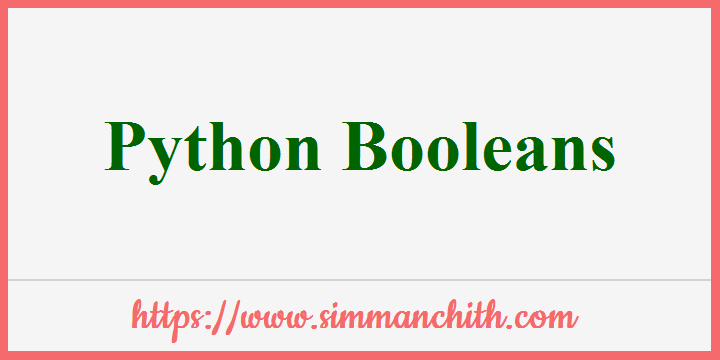# Python BooleansBooleans have two possible values which is, True or False.

You can also search for these topics, python booleans in list, python booleans type or not, list the booleans using python, assign the python booleans, define the arguments in python booleans and condition, what is python boolean algebra, how to sort by boolean in python, way to comparison of boolean in python, define the contants for python boolean.

## Boolean Values

It's common in programming to need to know whether an expression is `True` or `False`.

In Python, you can evaluate a certain expression and receive one of two results: `True` or `False`.

Example 1 :- Compare two values by evaluating the expression and by returning the boolean answer to Python :

``````print(10 > 9)
print(10 == 9)
print(10 < 9)``````

Output :-

True
False
False

Python will return `True` or `False` if you have a condition in a statement `if` :

Example 2 :- Depending on whether the condition is `True` or `False`, print the following message :

``````a = 200
b = 33
if b > a:
print("b is greater than a")
else:
print("b is not greater than a")``````

Output :-

b is not greater than a

You can also search for these topics, python returning boolean values, python boolean multiple values, python parser boolean values, value of none, boolean value of empty list in python, what is a boolean value in python, what is default value of boolean in python, what are the two boolean value in python, Example for python boolean values.

## Evaluate Values and Variables

You can evaluate any value by using the `bool()` function and return `True` or `False`.

Example 1 :- Evaluate a string and a number :

``````print(bool("Hello"))
print(bool(15))``````

Output :-

True
True

Example 2 :- Evaluate two variables :

``````x = "Hello"
y = 15
print(bool(x))
print(bool(y))``````

Output :-

True
True

You can also search for these topics, python evaluate value and variables type, how to evaluate values and variable in assignment default, boolean to evaluate values and variables in python, python values and variables by reference, python evaluate math expression without eval with values and variables, Example for values and variables in python.

## Most Values are True

If you have some sort of content, almost every value is evaluated to `True`.

Every string, except empty strings, is `True`.

Any number is `True`, except `0`.

Except for empty lists, tuples, sets and dictionaries, all are `True`.

Example :- The following will return True :

``````print(bool("abc"))
print(bool(123))
print(bool(["apple", "cherry", "banana"]))``````

Output :-

True
True
True

You can also search for these topics, python most value are true all list, check the most boolean values are true, how to check all values are true, check it all list most values are true in python, example for python most values are true.

## Some Values are False

In fact, with the exclusion of empty values (for instance `()`, `[]`, `{}`, `""` number 0 and value None), False does not value many values. And `False` value is of course `False` value.

Example 1 :- The following will return False :

``````print(bool(False))
print(bool(None))
print(bool(0))
print(bool(""))
print(bool(()))
print(bool([]))
print(bool({}))``````

Output :-

False
False
False
False
False
False
False

You can also search for these topics, python some values are false or not, boolean some values are false using python, list from the vslues are false using python, get the python some values are false, key values are false in python, check the working of Some Values are False using python, Example for python some values are false.

## Functions can Return a Boolean

The functions you can create can return a boolean value :

Example 1 :- Print the answer of a function :

``````def myFunction() :
return True
print(myFunction())``````

Output :-

True

You can run code based on a function's Boolean result :

Python has also many incorporated functions, such as the `isinstance()` function, that can be employed to determine if an object is of a certain type of data :

Example 2 :- Check if an object is an integer or not :

``````x = 200
print(isinstance(x, int))``````

Output :-

True

You can also search for these topics, python function can return a boolean example, type of Functions can Return a Boolean in python, check the value of return the boolean function using python, python check the function to return a boolean, python function can return boolean, python function can return a boolean false, Example for python functions can return a boolean.